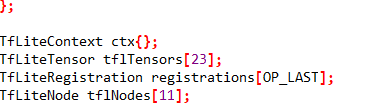# Some problems about deploment C++ library code

Hi!
I finished a keyword spotting application with edge impulse, which includes process of mfcc module, NN classifier(use 1D default neural network), and then I wanna deploy the model into C++ library.

When I try to read C++ library code, I encount some problems that I don’t understand.
First, why the number of tensors is 23? Can it be changed?How to change it ?
Second, what is tensor node, why the number of it is 11?
finally, I found that the tensor data are quantified, How did they quantify? Full integer quantization?Thank you so much!

Hi @sherry the number of nodes and tensors is dependent on the size of your neural network. You should not change the code that is generated, but if you create a smaller neural network or remove / add layers you’ll see these change.

Second, we use int8 quantization (default in TensorFlow since v2).

1 Like

Thanks so much!
Is the int8 quantization a method of tensorflow lite? Or is it another quantitative method designed by edge impulse?

We use normal TensorFlow Lite int8 quantization.

I just found out that the files deployed in C++ library have changed and the tflite-trained.cpp become all hexadecimal arrays, right?

Hi @sherry it depends on whether you run the quantized or unoptimized model. Quantized is `int8` arrays (mostly), unoptimized is `float` arrays. We haven’t changed anything here in the past weeks!